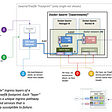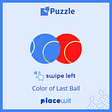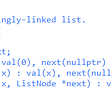# Dynamic Programming Patterns to Ace Interviews

## Recursion Memoization

• Visualise the problem as a tree
• Implement the tree using recursion
• Test it
• Add a base case to return memo values

## Array Tabulation

• TODO for later time :)
• Return one final value
• Decision Problem (True/False)
• Find a combination that matches given requirements
• Finding a optimised solution in the combination
• Remember the base case return type and final return should be the same
• When one of the inputs happened to be list of values, then there is gonna be a `for...loop`in the recursion
• When `for...loop` is involved, visualise what happens the last leaf nodes and build the result backwards

# Fibonacci Sequence

`Base cases:n < 0: Return nfib(n) : fib(n-1) + fib(n-2)`
`def fib(n, memo = {}):    if n in memo:        return memo[n]    if n <= 1:        return n    else:        # calculate for first time        memo[n] = fib(n-1, memo) + fib(n-2, memo)        return memo[n]`

# Grid Traveller

`Base cases:m or n ≤ 0 : When there is no cell to move return 0m and n = 1 : When there is only one cell return 1memo(m,n) = memo(m-1, n) + memo(m, n-1) `
`def grid_travel(m,n, memo={}):    """    :param m: Total number of rows    :param n: Total number of columns    :return:    """        # make the key with m & n, to differentiate rows and cols    key = f"m{m}n{n}"    if key in memo:        return memo[key]    if (m == 1 and n==1):        return 1    elif (m ==0 or n ==0):        return 0    else:        memo[key] = grid_travel(m-1, n, memo) + grid_travel(m, n-1, memo)        return memo[key]`

## canSum

`def can_sum(target_sum, values, memo={}):    """:param target_sum:    :param values: list    :return:    """    # print(memo, values)    if target_sum in memo:        return memo[target_sum]    if target_sum == 0:        return True    if target_sum < 0:        return False    for value in values:        reminder = target_sum - value        if can_sum(reminder, values, memo):            memo[target_sum] = True            return True     # If no way leads to zero then there is no possible values leads to sum    memo[target_sum] = False    return False`

## Can Construct

`def can_construct(target, word_bank, memo={}):   if target in memo:        return memo[target]   if target == '':        return True # When we found a way reconstruct the string   # Return true if any of branch returns true    for word in word_bank:        # check for matching prefix        if target.startswith(word):            suffix = target[len(word):]            if can_construct(suffix, word_bank):                memo[suffix] = True                return True    # If no branch leads to positive case, return false    memo[target] = False    return False`

## How Sum

`def how_sum(target_sum, values, memo={}):    if target_sum in memo:        return memo[target_sum]    if target_sum == 0:        return []    if target_sum < 0:        return None    for value in values:        ret = how_sum(target_sum - value, values, memo)        if ret is not None:            ret.append(value)            memo[target_sum] = ret            return ret    memo[target_sum] = None    return None`

## Count Construct

`def count_contruct(target, word_bank, memo={}):  if target in memo:    return memo[target]  if len(target) == 0 :    return True  total_ways = 0  for word in word_bank:    if target.startswith(word):      suffix = target[len(word):]      total_ways += count_contruct(suffix, word_bank)        memo[target] = total_ways  return total_ways`

## Best Sum

`def best_sum(target_sum, values, memo):  if target_sum in memo:        return memo[target_sum]  if target_sum == 0:        return []  if target_sum < 0:        return None  best_pair = None  for value in values:        ret = best_sum(target_sum=target_sum - value, values=values, memo=memo)        if ret is not None:            res = ret + [value]            if best_pair is None or len(res) < len(best_pair):                best_pair = res                memo[target_sum] = best_pair                # return best_pair # Dont return the value until all possible combinations are evaluated for child tree  memo[target_sum] = best_pair    return memo[target_sum]`

## All Construct

`def all_construct(target, word_bank, memo={}):    if target in memo:        return memo[target]    if target in memo:        return memo[target]    if target == '':        return [[]]    res = []    for word in word_bank:        if target.startswith(word):            suffix = target[len(word):]            suffix_ways = all_construct(suffix, word_bank)            target_ways = list(map(lambda l: l + [word], suffix_ways))            res.extend(target_ways)     memo[target] = res     return res`

--

--

--

## More from Mageswaran D

A simple guy in pursuit of of AI and Deep Learning with Big Data tools :) @ https://www.linkedin.com/in/mageswaran1989/

Love podcasts or audiobooks? Learn on the go with our new app.

## From SaaS to Studio: 3 Reasons to Make the Transition, the New Democratization of Mobile App…## Cloud Native Revolution## Docker Swarm Traefik state analysis## Node Reward Governance Vote Completed## First Experience with PostgreSQL 13 Beta 1## Mageswaran D

A simple guy in pursuit of of AI and Deep Learning with Big Data tools :) @ https://www.linkedin.com/in/mageswaran1989/

## Color of last ball II Puzzle## Linked List | LeetCode Top Interview Questions